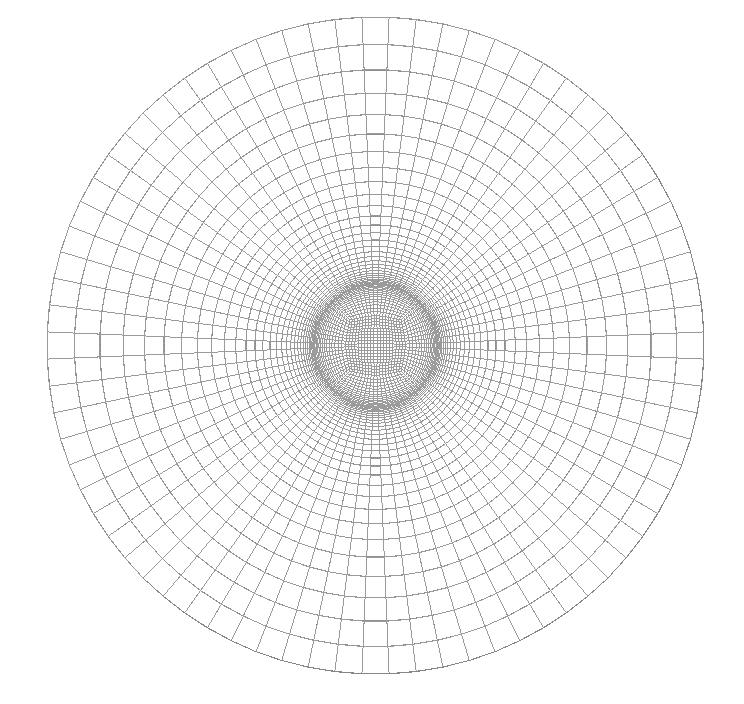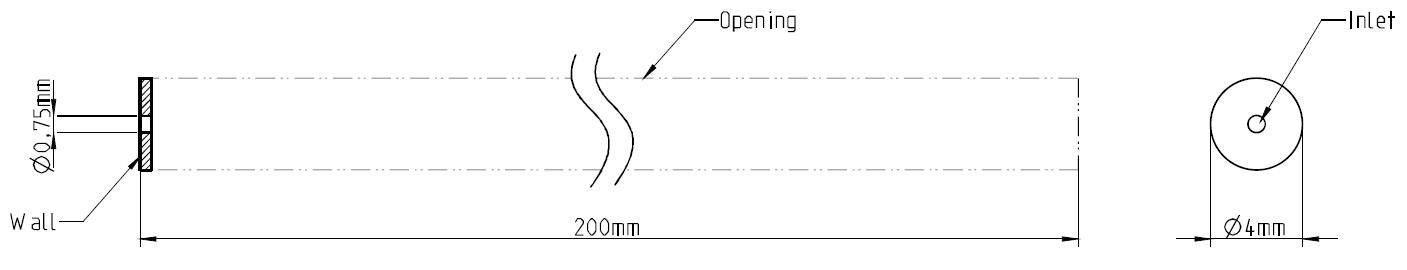# Simulation of a Water Spray

Hello everybody

I'm currently working on my Bachelor Thesis for my Mechanical Engineering degree.

The task is to simulate a water spray which is going to be injected into air as ideal gas. Both fluids need to be set as continuous fluid, so it should not be a large breakup model simulation to investigate every single drop. The nozzle diameter is 0.75mm and the injection velocity is 150 m/s.

I've already done my first setup but the result isn't quite comprehensibly. The water spray keeps cylindrical over a whole injection length of 200mm. I expect that the flow will spread after a time.

My question now is if someone has experience in modelling this kind of sprays? Do I need to add some special models or some other sort of physics? If you have some questions, please ask me!

Thanks for all replies in advance.

• edited May 2018

How did you come with the geometry for your simulation? And what are the boundary conditions? 2D or 3D? Can you provide a better schematic of the geometry and how you setup the BC's?

Which solver and what model are you using to solve this simulation?

CFD ANSYS Tutorial - Air jet flow simulation through a nozzle revisited | FLUENT

CFD Tutorial – Converging diverging (CD) nozzle supersonic flow | Fluent ANSYS

• Hi Rahul

I thought since the inlet is round, I'm going to do also a cylindrical opening. So I can create an ogrid (for the inlet) in an ogrid (for the opening). I meshed everything with hexa and did a refinement on the passage area between liquid and gas fluid. The mesh quality is really good and my supervisor is quite happy with it. So, everything is 3D, see pictures:My boundary conditions:
FLOW: Flow Analysis 1

• ANALYSIS TYPE:
EXTERNAL SOLVER COUPLING:
Option = None

• DOMAIN: Default Domain
Coord Frame = Coord 0
Domain Type = Fluid
Location = BODY
• BOUNDARY: Inlet
Boundary Type = INLET
Location = INLET
BOUNDARY CONDITIONS:

FLOW REGIME:
Option = Subsonic

HEAT TRANSFER:
Option = Fluid Dependent

MASS AND MOMENTUM:
Option = Cartesian Velocity Components
U = 0 [m s^-1]
V = 0 [m s^-1]
W = 150 m/s

TURBULENCE:
Option = Low Intensity and Eddy Viscosity Ratio

FLUID: Air
BOUNDARY CONDITIONS:

HEAT TRANSFER:
Option = Static Temperature
Static Temperature = 298 [K]

VOLUME FRACTION:
Option = Value
Volume Fraction = 0

FLUID: Water
BOUNDARY CONDITIONS:

HEAT TRANSFER:
Option = Static Temperature
Static Temperature = 298 [K]

VOLUME FRACTION:
Option = Value
Volume Fraction = 1
• BOUNDARY: Opening
Boundary Type = OPENING
Location = SIDE,BACK

BOUNDARY CONDITIONS:
FLOW DIRECTION:
Option = Normal to Boundary Condition

FLOW REGIME:
Option = Subsonic

HEAT TRANSFER:
Option = Fluid Dependent

MASS AND MOMENTUM:
Option = Opening Pressure and Direction
Relative Pressure = 1 [atm]

TURBULENCE:
Option = Low Intensity and Eddy Viscosity Ratio

FLUID: Air
BOUNDARY CONDITIONS:

HEAT TRANSFER:
Option = Static Temperature
Static Temperature = 298 [K]

VOLUME FRACTION:

FLUID: Water
BOUNDARY CONDITIONS:

HEAT TRANSFER:
Option = Static Temperature
Static Temperature = 298 [K]

VOLUME FRACTION:
• BOUNDARY: Wall
Boundary Type = WALL
Location = WALL

BOUNDARY CONDITIONS:
HEAT TRANSFER:

MASS AND MOMENTUM:
Option = Free Slip Wall
FLUID PAIR: Air | Water

BOUNDARY CONDITIONS:
Option = None
• DOMAIN MODELS:
BUOYANCY MODEL:
Option = Non Buoyant

DOMAIN MOTION:
Option = Stationary

MESH DEFORMATION:
Option = None

REFERENCE PRESSURE:
Reference Pressure = 0 [atm]

FLUID DEFINITION: Air
Material = Air Ideal Gas

MORPHOLOGY:
Option = Continuous Fluid

FLUID DEFINITION: Water
Material = Water
Option = Material Library

MORPHOLOGY:
Option = Continuous Fluid

FLUID MODELS:
COMBUSTION MODEL:
Option = None

FLUID: Air
HEAT TRANSFER MODEL:
Include Viscous Work Term = True
Option = Total Energy

FLUID: Water
HEAT TRANSFER MODEL:
Include Viscous Dissipation Term = On
Option = Thermal Energy

HEAT TRANSFER MODEL:
Homogeneous Model = Off
Option = Fluid Dependent

TURBULENCE MODEL:
Option = SST

TURBULENT WALL FUNCTIONS:
Option = Automatic

FLUID PAIR: Air | Water
Surface Tension Coefficient = 0.072 [N m^-1]

INTERPHASE HEAT TRANSFER:
Heat Transfer Coefficient = 10 [W m^-2 K^-1]
Option = Heat Transfer Coefficient

INTERPHASE TRANSFER MODEL:
Option = Free Surface

MASS TRANSFER:
Option = None

SURFACE TENSION MODEL:
Option = Continuum Surface Force
Primary Fluid = Water

MULTIPHASE MODELS:
Homogeneous Model = On

FREE SURFACE MODEL:
Option = Standard

INITIALISATION:
Option = Automatic

FLUID: Air

INITIAL CONDITIONS:
SET!

Thank you very much for the youtube tutorials! They seem quite useful! My only question is, how adaptable is it for CFX? Can I use it as a CFX user?

• Hi mrglobetrotter,

I have seen your question and project. I am working on same kind of project. can you please help me by sharing your work ( Maybe you have completed because your question is from 2018), this would be a great help to me. Thank you in advance

Sahil